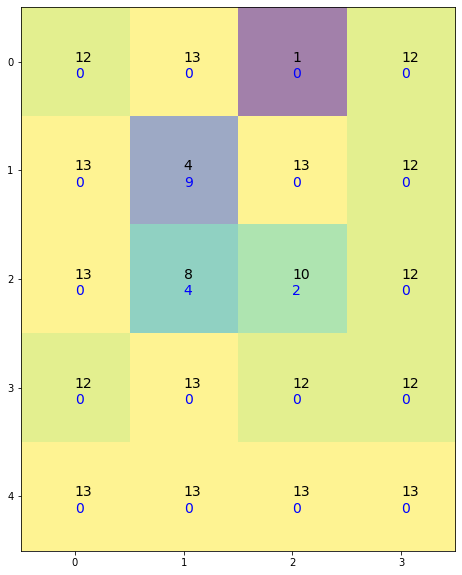Matpltlib 2D grid with labels

# Visualization Trapping Rainwater II - Leetcode 407

I’ve recently been working through some Leetcode problems and arrived at Trapping Rainwater II after completing Trapping Rainwater. By the way I really recommend For-All-Epsilon’s video on that problem. I was able to solve the question, with a hint from one of the first videos and I learnt a new technique from the third video.

Anyway, I was struggling to figure out why my naive solution wasn’t working so I built a quick visualization using `Matpltlib` to figure out what was going wrong.
Using a 2D grid with colour map and labels to show the elevation map and the water heights at each index.

## Matpltlib 2D Grid With Labels

``````import numpy as np
import matplotlib.pyplot as plt
from typing import List

# NOTE:
# - Function signature changed from Leetcode:
# `def trapRainWater(hm: List[List[int]]) -> int`  -> `def trapRainWater(hm: List[List[int]]) -> List[List[int]]:`
# - Algorithm for trapping rain is incorrect! The visualization helped me figure out why
def trapRainWater(hm: List[List[int]]) -> List[List[int]]:
m = len(hm)
if m < 3:
return 0
n = len(hm)
if n < 3:
return 0

total = 0
w = [*n for i in range(m)]
for i in range(m):
for j in range(n):
# min left right
lm = max(hm[i][:j+1])
rm = max(hm[i][j:])
lrm = min(lm, rm)
# min up down
dm = hm[i][j]
um = hm[i][j]
for k in range(i, m):
dm = max(dm, hm[k][j])
for k in reversed(range(0, i+1)):
um = max(um, hm[k][j])
w[i][j] = max(0, min(lrm, um, dm) - hm[i][j])
total += w[i][j]
return w
def plot_2d_grid_with_water(g):
w = trapRainWater(g)
fig = plt.figure(figsize=(10,10))
ax = plt.axes()

m = len(g)
n = len(g)

ax.axes.yaxis.set_ticks(range(m))
ax.axes.xaxis.set_ticks(range(n))

for i in range(m):
for j in range(n):
h = g[i][j]
label = f"{h}"
ax.text(j, i, label, fontsize=14)
ax.text(j, i+0.15, w[i][j], fontsize=14, color='blue')
plt.imshow(g, alpha=0.5)
plt.show()

lc = [[12,13,1,12],
[13,4,13,12],
[13,8,10,12],
[12,13,12,12],
[13,13,13,13]]
plot_2d_grid_with_water(lc)
``````## Why not 3D?

Originally I had planned to use a 3D bar plot for visualizing the elevation map as shown in the problem description, but I actually found it was more distracting and less clear than just doing a 2D grid with labels like this.